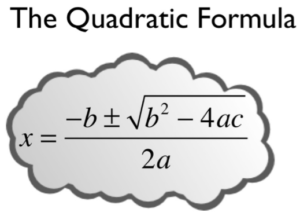### Learning Outcomes

• Write a quadratic equation in standard form and identify the values of $a$, $b$, and $c$ in a standard form quadratic equation.
• Use the Quadratic Formula to find all real solutions of a quadratic equation

You can solve any quadratic equation by completing the square—rewriting part of the equation as a perfect square trinomial. If you complete the square on the generic equation $ax^{2}+bx+c=0$ and then solve for $x$, you find that $x=\frac{-b\pm \sqrt{{{b}^{2}}-4ac}}{2a}$. This equation is known as the Quadratic Formula.We can derive the quadratic formula by completing the square. First, assume that the leading coefficient is positive; if it is negative, we can multiply the equation by $-1$ and obtain a positive a. Given $a{x}^{2}+bx+c=0$, $a\ne 0$, we will complete the square as follows:

1. First, move the constant term to the right side of the equal sign:
$a{x}^{2}+bx=-c$
2. As we want the leading coefficient to equal 1, divide through by a:
${x}^{2}+\frac{b}{a}x=-\frac{c}{a}$
3. Then, find $\frac{1}{2}$ of the middle term, and add ${\left(\frac{1}{2}\cdot\frac{b}{a}\right)}^{2}=\frac{{b}^{2}}{4{a}^{2}}$ to both sides of the equal sign:
${x}^{2}+\frac{b}{a}x+\frac{{b}^{2}}{4{a}^{2}}=\frac{{b}^{2}}{4{a}^{2}}-\frac{c}{a}$
4. Next, write the left side as a perfect square. Find the common denominator of the right side and write it as a single fraction:
${\left(x+\frac{b}{2a}\right)}^{2}=\frac{{b}^{2}-4ac}{4{a}^{2}}$
5. Now, use the square root property, which gives
$\begin{array}{l}x+\frac{b}{2a}=\pm \sqrt{\frac{{b}^{2}-4ac}{4{a}^{2}}}\hfill \\ x+\frac{b}{2a}=\frac{\pm \sqrt{{b}^{2}-4ac}}{2a}\hfill \end{array}$
6. Finally, add $-\frac{b}{2a}$ to both sides of the equation and combine the terms on the right side. Thus,
$x=\frac{-b\pm \sqrt{{b}^{2}-4ac}}{2a}$

This formula is very helpful for solving quadratic equations that are difficult or impossible to factor, and using it can be faster than completing the square. The Quadratic Formula can be used to solve any quadratic equation of the form $ax^{2}+bx+c=0$.

## Writing a Quadratic Equation in Standard Form

Remember that the form $ax^{2}+bx+c=0$ is called standard form of a quadratic equation. Before solving a quadratic equation using the Quadratic Formula, it is vital that you be sure the equation is in this form. If you do not, you might use the wrong values for a, b, or c, and then the formula will give incorrect solutions.

The following examples show how to ensure that your quadratic equation is in standard form and then correctly identify the values you will be using for a, b, and c in the Quadratic Formula.

### Example

Rewrite the equation $3x+2x^{2}+4=5$ in standard form and identify a, b, and c.

### Example

Rewrite the equation $2(x+3)^{2}–5x=6$ in standard form and identify a, b, and c.

### Try It

The Quadratic Formula will work with any quadratic equation, but only if the equation is in standard form, $ax^{2}+bx+c=0$. To use it, follow these steps.

1. Put the equation in standard form first.
2. Identify the coefficients, a, b, and c. Be sure to include negative signs if the bx or c terms are subtracted.
3. Carefully substitute the values noted in step $2$ into the equation. To avoid needless errors, use parentheses around each number input into the formula.
4. Simplify as much as possible.
5. Use the $\pm$ in front of the radical to separate the solution into two values: one in which the square root is added and one in which it is subtracted.
6. Simplify both values to get the possible solutions.

That is a lot of steps. Let us try using the Quadratic Formula to solve a relatively simple equation first; then you will go back and solve it again using another factoring method.

### Example

Use the Quadratic Formula to solve the equation $x^{2}+4x=5$.

You can check these solutions by substituting $1$ and $−5$ into the original equation.

 $\begin{array}{r}x=1\\x^{2}+4x=5\\\left(1\right)^{2}+4\left(1\right)=5\\1+4=5\\5=5\end{array}$ $\begin{array}{r}x=-5\\x^{2}+4x=5\,\,\,\,\,\\\left(-5\right)^{2}+4\left(-5\right)=5\,\,\,\,\,\\25-20=5\,\,\,\,\,\\5=5\,\,\,\,\,\end{array}$

You get two true statements, so you know that both solutions work: $x=1$ or $-5$. You have solved the equation successfully using the Quadratic Formula!

### Try it

Sometimes, it may be easier to solve an equation using conventional factoring methods like finding number pairs that sum to one number (in this example, $4$) and that produce a specific product (in this example $−5$) when multiplied. The power of the Quadratic Formula is that it can be used to solve any quadratic equation, even those where finding number combinations will not work.

In our next two video examples, we will see, first, a quadratic equation with two real, rational solutions and, second, a quadratic equation that has irrational solutions and that could not have been solved using factoring.

In the next video example, we show that the quadratic formula is useful when a quadratic equation has two irrational solutions that could not have been obtained by factoring.

Most of the quadratic equations you have looked at have two solutions, like the one above. The following example is a little different.

### Example

Use the Quadratic Formula to solve the equation $x^{2}-2x=6x-16$.

Again, check using the original equation.

$\begin{array}{r}x^{2}-2x=6x-16\,\,\,\,\,\\\left(4\right)^{2}-2\left(4\right)=6\left(4\right)-16\\16-8=24-16\,\,\,\,\,\,\\8=8\,\,\,\,\,\,\,\,\,\,\,\,\,\,\,\,\,\,\,\,\,\,\end{array}$

In the following video we show an example of using the quadratic formula to solve a quadratic equation that has one repeated solution.

In the next example, we will show that some quadratic equations do not have real solutions.  As we simplify with the quadratic formula, we may end up with a negative number under a square root, which, as we know, is not defined for real numbers.

### Example

Use the Quadratic Formula to solve the equation $x^2+x=-x-3$

### Summary

The Quadratic Formula is a useful way to solve any quadratic equation. The Quadratic Formula, $x=\frac{-b\pm \sqrt{{{b}^{2}}-4ac}}{2a}$, is found by completing the square of the quadratic equation . When you simplify using the quadratic formula and your result is a negative number under a square root, there are no real number solutions to the equation.

## Contribute!

Did you have an idea for improving this content? We’d love your input.